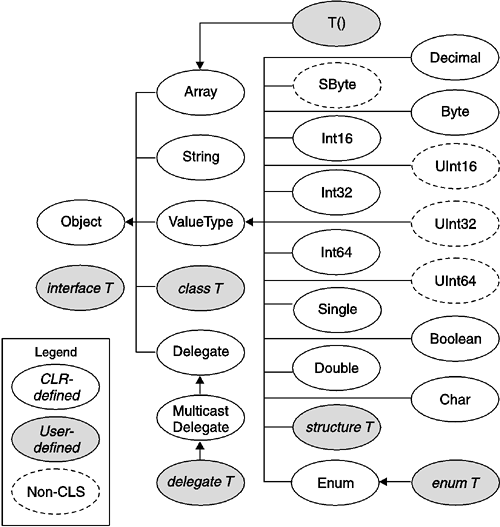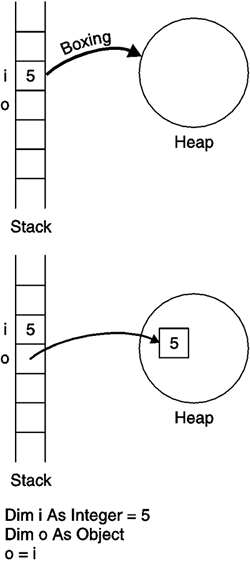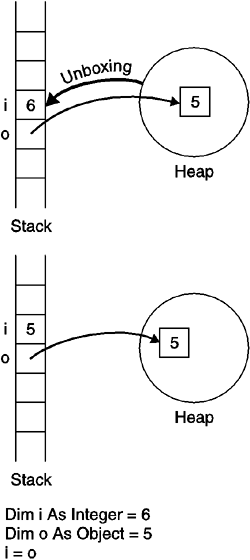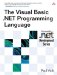# The .NET Framework Type Hierarchy

 <  Day Day Up  >

As previously discussed, if a class does not have an explicitly stated base class, its base class is Object . This means that all classes ultimately derive from Object . Indeed, all types in the Framework type system ”even the fundamental types, structures, enumerations, delegates, and arrays ”derive from Object through special base classes that cannot otherwise be inherited from (see Figure 13-2). Structures and the predefined types derive from the type System.ValueType . Enumerations derive from the type System.Enum . Delegates derive from the type System.Delegate . Arrays derive from the type System.Array . And all these types inherit from Object .

##### Figure 13-2. The .NET Framework Type HierarchyWhat this means is that any type in the type system can be converted to Object . This makes Object a universal type . A method that takes Object can accept any type, while a field typed as Object can store any type.

## Compatibility

The Object type combines the capabilities that used to be split between the Object type and the Variant type in previous versions of Visual Basic.

One interesting aspect of this design is that Object is a reference type. This raises the question: How can structures and fundamental types like Integer and Double , all of which are value types, inherit from a reference type? More specifically , how can a value type like Integer be converted to its base class, Object , when Object is a reference type? The Framework solves this conundrum through a process called boxing . When a value type is converted to Object , the Framework copies the value stored in the value type to the heap and returns a reference to the value. This process is called boxing the value type (see Figure 13-3). The reference can then be used to access the boxed value on the heap.

##### Figure 13-3. Boxing an Integer ValueWhen a reference to a boxed value type is converted back to the value type, the Framework copies the value stored on the heap back into the variable. This process is called unboxing a boxed value type (see Figure 13-4).

##### Figure 13-4. Unboxing an Integer ValueThe following code shows an example of boxing and unboxing an Integer .

` Module Test   Sub Main()     Dim o As Object     Dim i As Integer     i = 5     o = i  ' Copies the value to the heap     Console.WriteLine(o)     i = CInt(o)  ' Copies the value back from the heap     Console.WriteLine(i)   End Sub End Module `

#### DirectCast

In general, a boxed value type can only be unboxed back to its specific type. For example, the following code will throw an exception because a boxed value of structure X cannot be unboxed into a variable typed as structure Y .

` Structure X   Public Value As Integer End Structure Structure Y   Public Value As Integer End Structure Module Test   Sub Main()     Dim o As Object     Dim x As X     Dim y As Y     ' Box the value of x     o = x     ' Error: Cannot unbox a value of type X into a variable of type Y     y = CType(o, Y)   End Sub End Module `

The exceptions to this rule are the fundamental types: It is possible to unbox a boxed fundamental type into any other fundamental type that it has a conversion to. For example, the following code is valid because the Integer value in x can be unboxed into the Long variable y .

` Module Test   Sub Main()     Dim o As Object     Dim x As Integer = 5     Dim y As Long     ' Box the value of x     o = x     ' OK: Can unbox the Integer value into a Long variable     y = CLng(o)   End Sub End Module `

This can be very useful, but it comes at a price. When any value is converted from Object , the program must check at runtime to see whether the value is a boxed fundamental type so that it can apply the special unboxing behavior described in the previous paragraph. These checks add a little bit of overhead to the conversion, which normally is not significant. However, if it is known ahead of time that the conversion type exactly matches the boxed type, there may be some advantage to avoiding the overhead. For example, when lots of conversions are being performed, the overhead could become significant.

The DirectCast operator works just like the CType operator, except that it does not allow unboxing a boxed value type into anything but its original type ”even if the boxed value type is a fundamental type. The advantage, though, is that the overhead of checking for the fundamental types is avoided. For example, in the following code the second conversion will be more efficient than the first.

` Module Test   Sub Main()     Dim o As Object     Dim x As Integer = 5     Dim y, z As Integer     Dim a As Long     ' Box the value of x     o = x     ' Normal conversion     y = CInt(o)     ' More efficient conversion     z = DirectCast(o, Integer)     ' Error: Types do not match     a = DirectCast(o, Long)   End Sub End Module `

The last conversion emphasizes the fact that DirectCast can only unbox boxed values to their original type. So, unlike in the previous example, o cannot be unboxed into a Long variable.

## Style

Unless code is particularly performance sensitive and doing a lot of unboxing, CType is more general than DirectCast and is preferred.

 <  Day Day Up  >The Visual Basic .NET Programming Language
ISBN: 0321169514
EAN: 2147483647
Year: 2004
Pages: 173
Authors: Paul Vick

Similar book on Amazon